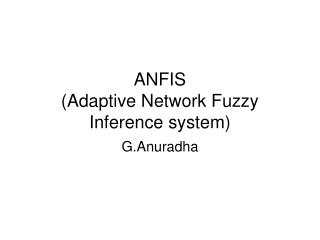Download PresentationANFIS (Adaptive Network Fuzzy Inference system)

# ANFIS (Adaptive Network Fuzzy Inference system) - PowerPoint PPT Presentation

ANFIS (Adaptive Network Fuzzy Inference system). G.Anuradha. Introduction. Conventional mathematical tools are quantitative in nature They are not well suited for uncertain problems FIS on the other hand can model qualitative aspects without employing precise quantitative analyses.I am the owner, or an agent authorized to act on behalf of the owner, of the copyrighted work described.
Download Presentation## ANFIS (Adaptive Network Fuzzy Inference system)

Download Policy: Content on the Website is provided to you AS IS for your information and personal use and may not be sold / licensed / shared on other websites without getting consent from its author.While downloading, if for some reason you are not able to download a presentation, the publisher may have deleted the file from their server.

- - - - - - - - - - - - - - - - - - - - - - - - - - E N D - - - - - - - - - - - - - - - - - - - - - - - - - -
Presentation Transcript
1. Introduction • Conventional mathematical tools are quantitative in nature • They are not well suited for uncertain problems • FIS on the other hand can model qualitative aspects without employing precise quantitative analyses. • Though FIS has more practical applications it lack behind • Standard methods for transformation into rule base • Effective methods for tuning MFs for better performance index

2. So…… ANFIS serve as a basis for constructing a set of fuzzy if-then rules with appropriate membership functions to generate the stipulated input-output pairs

3. Fuzzy if-then rules and Fuzzy Inference systems • Fuzzy if-then rules are of the form IF A THEN B where A and B are labels of fuzzy sets. • Example • “if pressure is high then volume is small” Linguistic variables Linguistic values

4. Sugeno model Assume that the fuzzy inference system has two inputs x and y and one output z. A first-order Sugeno fuzzy model has rules as the following: Rule1: If x is A1 and y is B1, then f1 = p1x + q1y + r1 Rule2: If x is A2 and y is B2, then f2 = p2x + q2y + r2

5. Fuzzy Inference system

6. Blocks of FIS

7. Steps of fuzzy reasoning

8. Types of fuzzy reasoning

9. Type 1: The overall output is the weighted average of each rule’s firing strength and output membership functions. • Type 2: The overall output is derived by applying the “max” operation to the qualified fuzzy outputs. The final crisp output can be obtained using some defuzzification methods • Type 3: Takegi and Sugeno fuzzy if-then rules are used. The output of each rule is a linear combination of input variables plus a constant term and the final output is the weighted average of each rule’s output

10. Adaptive Networks – Architecture and Learning Has parameters Has no parameters

11. Adaptive Networks – Architecture and Learning • Superset of all feedforward NN with supervised learning capability • Has nodes and directional links connecting different nodes • Part or all the nodes are adaptive(each output of these nodes depends on parameters pertaining to this node) and learning rule specifies how these parameters should be changed to minimize a error measure

12. Learning rule • The basic learning rule is gradient descent and chain rule • Because of the problem of slowness and being trapped in local minima a hybrid learning rule is proposed • This learning rule comes in two modes • Batch learning • Pattern learning

13. Architecture and basic learning • An adaptive network is a multi-layer feedforward network in which each node performs a particular function on the incoming signals • The nature and the choice of the node function depends on the overall input-output function • No weights are associated with links and the links just indicate the flow

14. Architecture and basic learning Contd… • To achieve desired i/p-o/p mapping the parameters are updated according to training data and gradient-based learning procedure

15. Gradient based learning procedure • Given adaptive network has L layers • k-th layer has #k nodes • (k,i)- ith node in the kth layer Node function- ith node in the k-layer Node output depends on its incoming signals and its parameter set and a,b,c etc. are parameters pertaining to this node

16. Learning paradigms for Adaptive networks • Batch learning:-Update action takes place only after the whole training data set has been presented(After an epoch) • On-line learning:-parameters are updated immediately after each input-output pair has been presented.

17. Hybrid Learning Rule-Batch-Off line learning rule • Combines gradient method and least square estimator to identify parameters Where I is a set of input variables and S is the set of parameters If there exists a function H such that the composite function HoF is linear in some of the elements of S, then these elements can be identified by the least square Method.

18. Using least square estimator we have For systems with changing characteristics, X can be iteratively calculated with the formulae given below. Usually used for online version Si is the covariance matrix. The initial conditions to the equation are X0=0 and where is a positive large number and I is the identity matrix

19. ANFIS(Adaptive Network based fuzzy inference system) • It is functionally equivalent to FIS • It has minimum constraints so very popular • It should be feedforward and piecewise differentiable